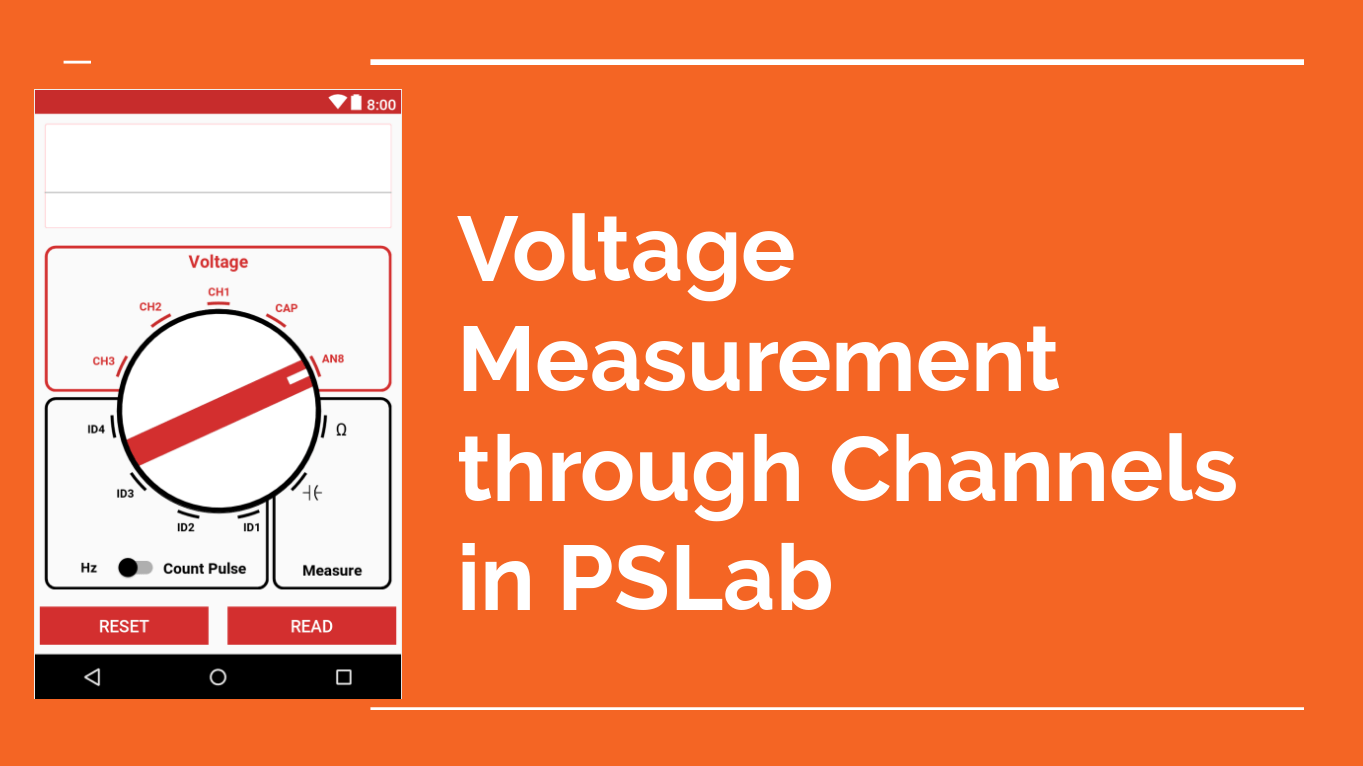# Voltage Measurement through Channels in PSLab

The Pocket Science Lab multimeter has got three channels namely CH1,CH2 and CH3 with different ranges for measuring the voltages.This blog will give a brief description on how we measure voltages in channels.

Measuring Voltages at channels can be divided into three parts:-

1. Communication between between device and Android.
2. Setting up analog channel (analog constants)
3. Voltage measuring function of android.

Communication between PSLab device and Android App

The communication between the PSLab device and  Android occurs through the help of UsbManger package of CommunicationHandler class of the app. The main two functions involved in the communication are read and write functions in which we send particular number of bytes and then we receive certain bytes.

```public int read(byte[] dest, int bytesToBeRead, int timeoutMillis) throws IOException {
} else {
}
}
//}
}```

Similarly the write function is –

```public int write(byte[] src, int timeoutMillis) throws IOException {
if (Build.VERSION.SDK_INT < 18) {
return writeSupportAPI(src, timeoutMillis);
}
int written = 0;
while (written < src.length) {
int writeLength, amtWritten;
//synchronized (mWriteBufferLock) {
writeLength = Math.min(mWriteBuffer.length, src.length - written);
// bulk transfer supports offset from API 18
amtWritten = mConnection.bulkTransfer(mWriteEndpoint, src, written, writeLength, timeoutMillis);
//}
if (amtWritten < 0) {
throw new IOException("Error writing " + writeLength +
" bytes at offset " + written + " length=" + src.length);
}
written += amtWritten;
}
return written;
}```

Although these are the core functions used for communication but the data received through these functions are further processed using another class known as PacketHandler. In the PacketHandler class also there are two major functions i.e sendByte and getByte(), these are the main functions which are further used in other classes for communication.

The sendByte function:-

```public void sendByte(int val) throws IOException {
if (!connected) {
throw new IOException("Device not connected");
}
try {
mCommunicationHandler.write(new byte[] {
(byte)(val & 0xff), (byte)((val >> 8) & 0xff)
}, timeout);
} catch (IOException e) {
Log.e("Error in sending int", e.toString());
e.printStackTrace();
}
} else {
burstBuffer.put(new byte[] {
(byte)(val & 0xff), (byte)((val >> 8) & 0xff)
});
}
}```

As we can see that in this function also the main function used is the write function of communicationHandler but in this class the data is further processed.

Setting Up the Analog Constants

For setting up the ranges, gains and other properties of channels, a different class of AnalogConstants is implemented in the android app, in this class all the properties which are used by the channels are defined which are further used in the sendByte() functions for communication.

```public class AnalogConstants {

public double[] gains = {1, 2, 4, 5, 8, 10, 16, 32, 1 / 11.};
public String[] allAnalogChannels = {"CH1", "CH2", "CH3", "MIC", "CAP", "SEN", "AN8"};
public String[] biPolars = {"CH1", "CH2", "CH3", "MIC"};
public Map<String, double[]> inputRanges = new HashMap<>();
public Map<String, Integer> picADCMultiplex = new HashMap<>();

public AnalogConstants() {

inputRanges.put("CH1", new double[]{16.5, -16.5});
inputRanges.put("CH2", new double[]{16.5, -16.5});
inputRanges.put("CH3", new double[]{-3.3, 3.3});
inputRanges.put("MIC", new double[]{-3.3, 3.3});
inputRanges.put("CAP", new double[]{0, 3.3});
inputRanges.put("SEN", new double[]{0, 3.3});
inputRanges.put("AN8", new double[]{0, 3.3});

}
}
```

Also in the AnalogInput sources class many other properties such as CHOSA( a variable assigned to denote the analog to decimal conversion constant of each channel) are also defined

```public AnalogInputSource(String channelName) {
AnalogConstants analogConstants = new AnalogConstants();
this.channelName = channelName;
range = analogConstants.inputRanges.get(channelName);
gainValues = analogConstants.gains;
calPoly10 = new PolynomialFunction(new double[] {
0.,
3.3 / 1023,
0.
});
calPoly12 = new PolynomialFunction(new double[] {
0.,
3.3 / 4095,
0.
});
if (range - range < 0) {
inverted = true;
inversion = -1;
}
if (channelName.equals("CH1")) {
gainEnabled = true;
gainPGA = 1;
gain = 0;
} else if (channelName.equals("CH2")) {
gainEnabled = true;
gainPGA = 2;
gain = 0;
}
gain = 0;
regenerateCalibration();
}```

Also in this constructor a polynomial function is also called which further plays an important  role in measuring voltage as it is through this polynomial function we get the voltage of channels in the science lab class , also it is also used in oscilloscope for plotting the graph . So this was the setup of analog channels.

Voltage Measuring Functions

There are two major functions for measuring voltages which are present in the scienceLab class

• getAverageVoltage
• getRawableVoltage

Here are the functions

```private double getRawAverageVoltage(String channelName) {
try {
int chosa = this.calcCHOSA(channelName);
mPacketHandler.sendByte(mCommandsProto.GET_VOLTAGE_SUMMED);
mPacketHandler.sendByte(chosa);
int vSum = mPacketHandler.getVoltageSummation();
mPacketHandler.getAcknowledgement();
return vSum / 16.0;
} catch (IOException | NullPointerException e) {
e.printStackTrace();
Log.e(TAG, "Error in getRawAverageVoltage");
}
return 0;
}```

This is the major function which takes the data from the communicationHandler class via packetHandler. Further this function is used in the getAverageVoltage function.

```private double getAverageVoltage(String channelName, Integer sample) {
if (sample == null) sample = 1;
PolynomialFunction poly;
double sum = 0;